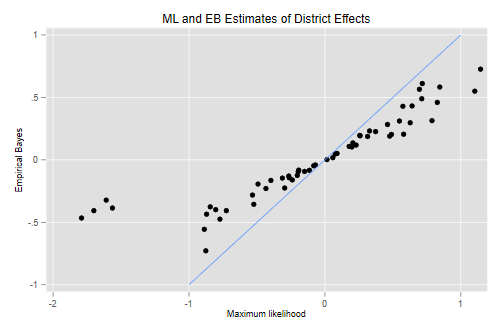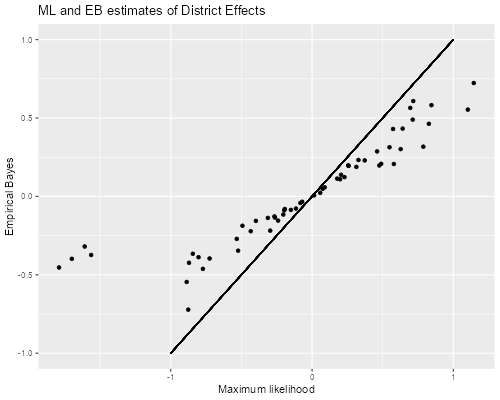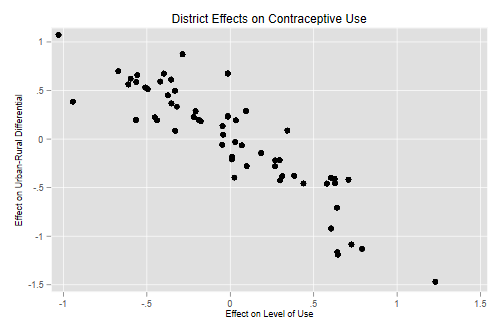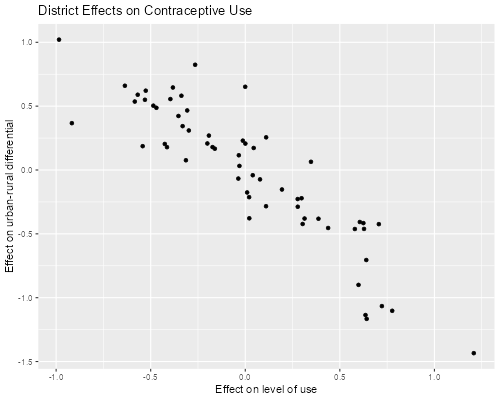Germán Rodríguez
Multilevel Models Princeton UniversityThe Stata manual [ME, p. 164] analyzes data from the 1989 Bangladesh fertility survey, see Huq and Cleland (1990), also analyzed by Ng et al. (2006).

```. webuse bangladesh, clear

. desc

Observations:         1,934                  Bangladesh Fertility Survey, 1989
Variables:             8                  28 May 2020 20:27
(_dta has notes)
────────────────────────────────────────────────────────────────────────────────
Variable      Storage   Display    Value
name         type    format    label      Variable label
────────────────────────────────────────────────────────────────────────────────
district        byte    %9.0g                 District
c_use           byte    %9.0g      yesno      Use contraception
urban           byte    %9.0g      urban      Urban or rural
age             float   %6.2f                 Age, mean centered
child1          byte    %9.0g                 1 child
child2          byte    %9.0g                 2 children
child3          byte    %9.0g                 3 or more children
children        byte    %18.0g     childlbl   Number of children
────────────────────────────────────────────────────────────────────────────────
Sorted by: district
```
```> library(haven)
> names(bd)
 "district" "c_use"    "urban"    "age"      "child1"   "child2"   "child3"
```

### A Random-Intercept Model

We will fit a logit model predicting contraceptive use by urban residence, age and number of children, with a random intercept by district. We use `melogit`, which allows us to obtain estimates of the district effect, and supersedes `xtmelogit` and `meqrlogit` as of Stata 16.

```. melogit c_use urban age child1 child2 child3 || district:

Fitting fixed-effects model:

Iteration 0:   log likelihood = -1229.5485
Iteration 1:   log likelihood = -1228.5268
Iteration 2:   log likelihood = -1228.5263
Iteration 3:   log likelihood = -1228.5263

Refining starting values:

Grid node 0:   log likelihood = -1219.2681

Fitting full model:

Iteration 0:   log likelihood = -1219.2681  (not concave)
Iteration 1:   log likelihood = -1207.5978
Iteration 2:   log likelihood = -1206.8428
Iteration 3:   log likelihood = -1206.8322
Iteration 4:   log likelihood = -1206.8322

Mixed-effects logistic regression               Number of obs     =      1,934
Group variable: district                        Number of groups  =         60

Obs per group:
min =          2
avg =       32.2
max =        118

Integration method: mvaghermite                 Integration pts.  =          7

Wald chi2(5)      =     109.60
Log likelihood = -1206.8322                     Prob > chi2       =     0.0000
─────────────┬────────────────────────────────────────────────────────────────
c_use │ Coefficient  Std. err.      z    P>|z|     [95% conf. interval]
─────────────┼────────────────────────────────────────────────────────────────
urban │   .7322765   .1194857     6.13   0.000     .4980888    .9664641
age │  -.0264981   .0078916    -3.36   0.001    -.0419654   -.0110309
child1 │   1.116001   .1580921     7.06   0.000     .8061465    1.425856
child2 │   1.365895   .1746691     7.82   0.000      1.02355     1.70824
child3 │   1.344031   .1796549     7.48   0.000     .9919139    1.696148
_cons │   -1.68929   .1477591   -11.43   0.000    -1.978892   -1.399687
─────────────┼────────────────────────────────────────────────────────────────
district     │
var(_cons)│    .215618   .0733222                      .1107208    .4198954
─────────────┴────────────────────────────────────────────────────────────────
LR test vs. logistic model: chibar2(01) = 43.39       Prob >= chibar2 = 0.0000

. estimates store ri

. mata exp(st_matrix("e(b)"))
1             2             3             4             5
┌───────────────────────────────────────────────────────────────────────
1 │  2.079809828   .9738498479   3.052623679   3.919229121   3.834469354
└───────────────────────────────────────────────────────────────────────
6             7
─────────────────────────────┐
1     .184650664   1.240628421  │
─────────────────────────────┘
```

R’s `lme4` uses the Laplace approximation by default, but also lets us specify the number of adaptive quadrature points. We try both and compare estimates

```> library(lme4)
> ri <- glmer(c_use ~ urban + age + child1 + child2 + child3 +
+         (1 | district), data =bd, family=binomial)
> ri7 <- glmer(c_use ~ urban + age + child1 + child2 + child3 +
+         (1 | district), data =bd, family=binomial, nAGQ=7)
> cbind(fixef(ri), fixef(ri7))
[,1]        [,2]
(Intercept) -1.68878347 -1.68929490
urban        0.73283848  0.73228505
age         -0.02649254 -0.02649815
child1       1.11581705  1.11600499
child2       1.36570152  1.36589348
child3       1.34361785  1.34403031
> c(unlist(VarCorr(ri)), unlist(VarCorr(ri7)))
district  district
0.2124891 0.2156170
```

The estimates of the coefficients are practically the same, and the estimates of the variance of the intercepts are very similar. In my experience the Laplace approximation works much better for Poisson than for Binomial models.

The estimates show that when we compare women with the same age and family size, contraceptive use is higher in urban than in rural areas, with double the odds of using contraception (exp(0.732)=2.08). Within an area and family size, contraceptive use declines with age, with 2.6% lower odds per year of age (exp(-.026)-1 = -.026). Within an area and age, contraceptive use is higher among women with a child, and much higher among women with two or more children, than among those with no children, with odds ratios of three and almost four (exp(1.116)=3.05, exp(1.366)=3.92 and exp(1.344)=3.834).

There is very subtantial variation in contraceptive use across districts. The standard deviation of 0.46 indicates that women in a district which is one standard deviation above the mean have odds of using contraception that are 59% higher than comparable women in an average district (exp(0.464) = 1.59). The standard deviation is also equivalent to a correlation of 0.06 in the latent propensities to use contraception of comparable women in the same district (0.4642/(0.4642+ π2/3) = 0.06).

#### ML and Empirical Bayes Estimates

We can identify districts where women are more or less likely to use contraception by “estimating” the random effects. We can obtain maximum likelihood estimates by treating the estimated linear predictor from this model as an offset and then fitting a separate regression model in each district. This is easy to do with `statsby`, as shown by Rabe-Hesketh and Skrondal(2012, p. 544). `dplyr's`do()`, as shown below.

```. predict xb, xb

. statsby mle=_b[_cons], by(district) saving(ml,replace): ///
>     logit c_use, offset(xb)
(running logit on estimation sample)

Command: logit c_use, offset(xb)
mle: _b[_cons]
By: district

Statsby groups
────┼─── 1 ───┼─── 2 ───┼─── 3 ───┼─── 4 ───┼─── 5
..x.......x.....................................x.    50
..........

. merge m:1 district using ml

Result                      Number of obs
─────────────────────────────────────────
Not matched                             0
Matched                             1,934  (_merge==3)
─────────────────────────────────────────
```
```> library(dplyr)
> bd\$xb = model.matrix(ri7) %*% fixef(ri7)
> fits <- group_by(bd, district) |>
+     do( lf = glm(c_use ~ 1 + offset(xb), family=binomial, data= .))
> mles <- data.frame(district = fits[], mle= sapply(fits[], coef)) |>
+     filter(abs(mle) < 10) # all 0 or 1
```

The mle is not defined when all women in a district have the same outcome, so everybody (or nobody) uses contraception. This occurs for 3 districts.

The empirical Bayes estimates, based on the posterior distribution of the random effects, can be obtained with `predict, reffects`, which defaults to posterior means but can also calculate posterior modes by adding the option `ebmode`. `ranef()`, which calculates posterior modes.

```. estimates restore ri
(results ri are active now)

. predict re, ref
(calculating posterior means of random effects)

. bysort district: gen first=_n==1

. scatter re mle if first || function y=x, range(-1 1) ///
>   ,title(ML and EB Estimates of District Effects) ///
>   xtitle(Maximum likelihood) ytitle(Empirical Bayes) ///
>   legend(off)

. graph export bd1.png, width(500) replace
file bd1.png saved as PNG format
``````> library(ggplot2)
> re <- ranef(ri7)\$district
> ebs  <- data.frame(district=as.numeric(row.names(re)), eb=re[,1])
> ebms <- left_join(ebs, mles)
> ggplot(ebms, aes(mle, eb)) + geom_point() +
+     geom_segment(aes(x=-1,y=-1,xend=1,yend=1)) +
+     ggtitle("ML and EB estimates of District Effects") +
+     xlab("Maximum likelihood") + ylab("Empirical Bayes")
> ggsave("bd1r.png", width=500/72, height=400/72, dpi=72)
```Comparing the EB and ML estimates we see the usual shrinkage towards zero, which is particularly noticeable in four districts:

```. egen ni = count(1), by(district)

. egen pi = mean(c_use), by(district)

. list district ni pi mle re if first & mle < -1, clean

district   ni         pi         mle          re
352.         10   13   .0769231   -1.563583   -.3855997
764.         24   14   .0714286   -1.790981   -.4655188
1740.         55    6   .1666667   -1.609306   -.3224564
1851.         59   10         .1   -1.700366   -.4066908
```
```> ebmsp <- left_join(ebms, (group_by(bd, district) |>
+     summarize(n=n(), p=mean(c_use))))
> filter(ebmsp, mle < -1)
district         eb       mle  n          p
1       10 -0.3736197 -1.563578 13 0.07692308
2       24 -0.4534363 -1.790976 14 0.07142857
3       55 -0.3196332 -1.609310  6 0.16666667
4       59 -0.3979384 -1.700360 10 0.10000000
```

These are all fairly small districts with less than 15 women each. However, there are five other districts with small sizes that do not exhibit this phenomenon. The reason is that in those cases the estimated random effect was close to zero anyway. (There are also two small districts with all zeroes or all ones.)

```. list district ni pi mle re if first & ni < 15 & mle > -1, clean

district   ni         pi         mle          re
138.          3    2          1           .    .2077022
845.         26   13   .3846154    .0580263    .0169653
1101.         33   14   .4285714   -.1926461   -.0822259
1215.         37   13   .5384616    .7878245    .3139492
1228.         38   14   .2857143   -.4925295   -.1938299
1335.         42   11   .5454546    .5789949    .2049814
1600.         49    4          0           .    -.192383
```
```> filter(ebmsp, mle > -1 & n < 15)
district          eb         mle  n         p
1       26  0.02288111  0.05803125 13 0.3846154
2       33 -0.08019613 -0.19264557 14 0.4285714
3       37  0.31738383  0.78782993 13 0.5384615
4       38 -0.18712314 -0.49252888 14 0.2857143
5       42  0.20673628  0.57899497 11 0.5454545
```

#### Predicted Probabilities

Subject-specific probabilities can be computed easily from first principles by setting the fixed covariates and the random effects to illustrative values. In this dataset age is centered at the sample mean, which simplifies matters. Here are probabilities for a woman with one child and average age in urban and rural areas of the average district:

```. di invlogit(_b[_cons] + _b[child1])
.36047846

. di invlogit(_b[_cons] + _b[child1] + _b[urban])
.53966358
```
```> b <- fixef(ri7)
> xb <- b["(Intercept)"] + b["child1"]
> data.frame(rural=plogis(xb), urban = plogis(xb + b["urban"]))
rural     urban
(Intercept) 0.360478 0.5396653
```

We see that the odds ratio of about two translates in this case to a difference of 18 percentage points (between 36.0% and 54.0%).

Population-average probabilities can also be computed, but they require integration over the distribution of the random effect, which we do below from first principles using Gaussian quadrature. In Stata these probabilities can also be computed by using `gllapred` after `gllamm`. I wrote an R function to compute the quadrature points by translating the code in Numerical Recipes for C, Press et al. (1992).

Here’s the calculation of the rural and urban probabilities averaged over the distribution of the district effects. We list the estimates to find the location of the coefficients of interest: the constant is 6th, the urban coef is first, the one-child coef is 3rd, and the log of sigma is 7th.

```. mat list e(b)

e(b)[1,7]
c_use:         c_use:         c_use:         c_use:         c_use:

urban            age         child1         child2         child3
y1      .73227646     -.02649815      1.1160014       1.365895      1.3440311

c_use:             /:
var(
_cons  _cons[dis~t])
y1     -1.6892895      .21561804

. mata
───────────────────────────────────────────────── mata (type end to exit) ──────
:   gh = _gauss_hermite_nodes(12)'   // transpose

:   gh[,1] = gh[, 1] :* sqrt(2)      // change of variables

:   gh[,2] = gh[, 2] :/ sqrt(pi())   // to standard normal

:   b = st_matrix("e(b)")

:   sig = exp(b)

:   u = r = 0

:   for (i=1; i <= 12; i++) {
>     r = r + invlogit(b + b + sig*gh[i,1]) * gh[i,2]
>     u = u + invlogit(b + b + sig*gh[i,1] + b) * gh[i,2]
>   }

:   r, u
1             2
┌─────────────────────────────┐
1 │  .3915468493   .5304613607  │
└─────────────────────────────┘

: end
────────────────────────────────────────────────────────────────────────────────
```
```> source("https://grodri.github.io/multilevel/gauher.R")
> gh <- gauher(12) # z is abscissa and w is weight
> sig <- sqrt(unlist(VarCorr(ri7)))
> u <- r <- 0
> # recall xb = b["(Intercept)"] + b["child1"]
> for(i in 1:12) {
+     r <- r + plogis(xb + sig * gh[i,"z"]) * gh[i,"w"]
+     u <- u + plogis(xb + sig * gh[i,"z"] + b["urban"]) * gh[i,"w"]
+ }
> data.frame(rural=r, urban=u)
rural     urban
(Intercept) 0.3668264 0.5377387
```

The population-average effect of urban residence is a difference between 36.7% and 53.8% or 17.1 percentage points, averaged across all districts.

### A Random Slope Model

The next model will treat the urban-rural difference as random at the district level. (Most districts have urban and rural areas.) This is somewhat equivalent to allowing an interaction between urban residence and district, but instead of estimating a separate urban-rural difference for each district, we assume that the differences are drawn from a normal distribution:

```. melogit c_use urban age child1 child2 child3 ///
>   || district: urban, covariance(unstructured)

Fitting fixed-effects model:

Iteration 0:   log likelihood = -1229.5485
Iteration 1:   log likelihood = -1228.5268
Iteration 2:   log likelihood = -1228.5263
Iteration 3:   log likelihood = -1228.5263

Refining starting values:

Grid node 0:   log likelihood = -1215.8592

Fitting full model:

Iteration 0:   log likelihood = -1215.8592  (not concave)
Iteration 1:   log likelihood = -1201.0652
Iteration 2:   log likelihood = -1199.6394
Iteration 3:   log likelihood = -1199.3157
Iteration 4:   log likelihood =  -1199.315
Iteration 5:   log likelihood =  -1199.315

Mixed-effects logistic regression               Number of obs     =      1,934
Group variable: district                        Number of groups  =         60

Obs per group:
min =          2
avg =       32.2
max =        118

Integration method: mvaghermite                 Integration pts.  =          7

Wald chi2(5)      =      97.50
Log likelihood = -1199.315                      Prob > chi2       =     0.0000
─────────────┬────────────────────────────────────────────────────────────────
c_use │ Coefficient  Std. err.      z    P>|z|     [95% conf. interval]
─────────────┼────────────────────────────────────────────────────────────────
urban │   .8157875   .1715519     4.76   0.000     .4795519    1.152023
age │   -.026415    .008023    -3.29   0.001    -.0421398   -.0106902
child1 │    1.13252   .1603285     7.06   0.000      .818282    1.446758
child2 │   1.357739   .1770522     7.67   0.000     1.010723    1.704755
child3 │   1.353827   .1828801     7.40   0.000     .9953882    1.712265
_cons │   -1.71165   .1605618   -10.66   0.000    -2.026345   -1.396954
─────────────┼────────────────────────────────────────────────────────────────
district     │
var(urban)│   .6663237   .3224689                       .258074    1.720387
var(_cons)│   .3897448   .1292463                       .203473    .7465413
─────────────┼────────────────────────────────────────────────────────────────
district     │
cov(urban,│
_cons)│  -.4058861   .1755414    -2.31   0.021    -.7499408   -.0618313
─────────────┴────────────────────────────────────────────────────────────────
LR test vs. logistic model: chi2(3) = 58.42               Prob > chi2 = 0.0000

Note: LR test is conservative and provided only for reference.

. mata exp(st_matrix("e(b)"))
2.260955428
```

It is very important in this model to specify the covariance matrix of the random effects as unstructured, so we allow correlation between the level of contraceptive use and the urban-rural difference in each district.

```> rs <- glmer(c_use ~ urban + age + child1 + child2 + child3 +
+   (1 + urban | district), data =bd, family=binomial)
> rs
Generalized linear mixed model fit by maximum likelihood (Laplace
Approximation) [glmerMod]
Family: binomial  ( logit )
Formula: c_use ~ urban + age + child1 + child2 + child3 + (1 + urban |
district)
Data: bd
AIC       BIC    logLik  deviance  df.resid
2417.290  2467.396 -1199.645  2399.290      1925
Random effects:
Groups   Name        Std.Dev. Corr
district (Intercept) 0.6177
urban       0.8019   -0.80
Number of obs: 1934, groups:  district, 60
Fixed Effects:
(Intercept)        urban          age       child1       child2       child3
-1.71078      0.81508     -0.02641      1.13217      1.35775      1.35303
> exp(fixef(rs))
(Intercept)       urban         age      child1      child2      child3
0.1807254   2.2593497   0.9739367   3.1023807   3.8874183   3.8691167
```

Adaptive quadrature is not available in `glmer` for models with more than one random effect, so we rely on the Laplace approximation. The estimates are very similar to those obtained with Stata using adaptive quadrature, which are quoted below.

The estimates show that the odds of using contraception on the average district are about double in urban compared to urban areas, but the difference varies substantially by district, as shown by the standard deviation of the urban coefficient, 0.82. For example the urban-rural difference in logits is essentially zero in a district with differential one standard deviation below the mean (0.8158-0.8163 = -0.001), and equivalent to an odds ratio of about five in a district with differential one standard deviation above the mean (0.8158+0.8163 = 1.632).

#### EB Estimates of the Intercept and Slope

We now compute Empirical Bayes (EB) estimates of the random intercept and random slop, representing the estimated district effects on the leel of contraceptive use and the urban-rural difference. (We could compute ML estimates as well, using a procedure similar to the previous section, but we will focus on the EB estimates.)

```. predict rs ri, reffects  // Stata puts the intercept last
(calculating posterior means of random effects)

. scatter rs ri, title(District Effects on Contraceptive Use) ///
>   xtitle(Effect on Level of Use) ///
>   ytitle(Effect on Urban-Rural Differential)

. graph export bd2.png, width(500) replace
file bd2.png saved as PNG format
``````> eb = ranef(rs)\$district
> names(eb)= "level" # better than (Intercept)
> ggplot(eb, aes(level, urban)) + geom_point() +
+   ggtitle("District Effects on Contraceptive Use") +
+   xlab("Effect on level of use") +
+   ylab("Effect on urban-rural differential")
> ggsave("bd2r.png", width=500/72, height=400/72, dpi=72)
```We see a clear negative correlation. Districts where contraceptive use is higher than average, after considering the age and number of children of the residents, tend to show a much smaller urban-rural difference, reflecting the correlation of -0.796 estimated above.

We could also estimate separate random effects for urban and rural areas in each district by including dummies for both urban and rural and omitting the constant in the fixed and random parts. If you do that, you will find that the two effects are nearly independent.

Huq, N. M., and J. Cleland. (1990). Bangladesh Fertility Survey 1989 (Main Report). National Institute of Population Research and Training.

Ng, E. S.-W., J. R. Carpenter, H. Goldstein, and J. Rasbash. (2006). Estimation in generalised linear mixed models with binary outcomes by simulated maximum likelihood. Statistical Modelling 6: 23–42.

Press, W. H., S. A. Teukolsky, W. T. Vetterling, B. P. Flannery (1992). Numerical Recipes in C: The Art of Scientific Computing, Second Edition, Cambridge University Press.

Rabe-Hesketh, S and A. Skrondal (2012). Multilevel and Longitudinal Models Using Stata. Volume II: Categorical Responses, Counts and Survival. Third Edition. College Station, TX: Stata Press.

StataCorp (2021). Stata Multilevel Mixed-Effects Reference Manual. Release 17. College Station, TX: Stata Press.

